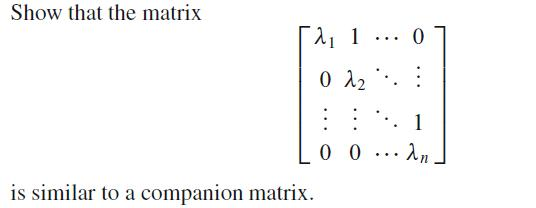# Show companion matrix is similar to the following matrix

## Homework Statement

need to show companion matrix is similar to the following matrix(here is the picture of the matrix)

## Homework Equations

here is the companion matrix
http://en.wikipedia.org/wiki/Companion_matrix

information on matrix similarity
http://en.wikipedia.org/wiki/Matrix_similarity

## The Attempt at a Solution

say given matrix is A and companion matrix is C then need to show

A = P^-1 * C * P for some invertible matrix P

i guess i could reduce guesswork by rewriting as

P*A = C*P

but even then it does not seem to be the ideal way to go about things.

Last edited:

## Answers and Replies

I don't think you put in the right link to the matrix...

oh lol fixed

What is the characteristic and minimal polynomial of that matrix? Can you construct the rational canonical form based off of elementary divisors?

i know char for companion but that is all.

i also know:

if same minimal poly then similar

if same frobenius canonical form then similar

but no clue how to go about finding them

Since your matrix is upper triangular, finding the characteristic polynomial is easy: It is just ##∏ (x-\lambda_i)##. If you can show that this is the minimal polynomial as well, then you are done.